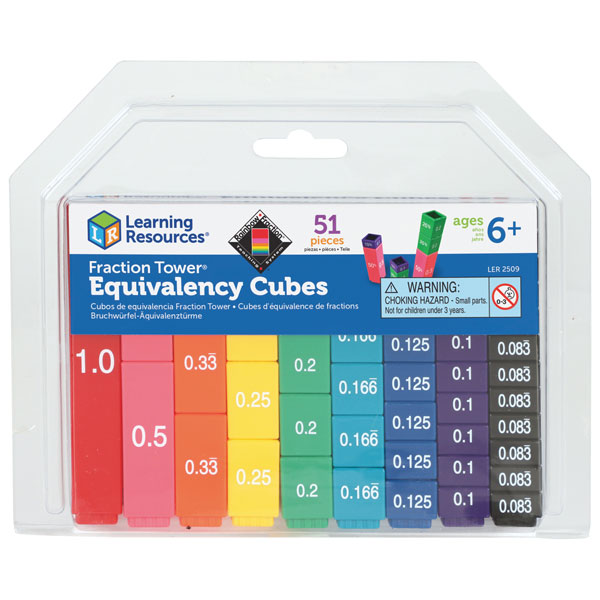Compare Prices - What are you looking for?# Learning Resources Fraction Tower Equivalency Set

Learning Resources Fraction Tower Fraction Cube Sets - Learning Resources LER2509, The Learning Resources Fraction Tower Cubes Equivalency Set is ideal for helping young maths learners to understand and visualise fraction concepts and equivalences by using the colour-coded pieces.The durable, interlocking cubes allow children to build simple, mathematical models. The set is perfect for developing a range of hands-on fraction and equivalency skills as well as being a useful maths accessory. Learning Resources Fraction Tower Equivalency Set

Price: £11.42 from Rapid Online Rapid Electronics Ltd.

Review This Product

Stockist Catalogue Product Name Price
Learning Resources Fraction Tower Equivalency Set £11.42

## Related Products

#### Learning Resources Fraction Tower Fraction Cubes

Learning Resources Fraction Tower Fraction Cube Sets - Learning Resources LER2510, The Learning Resources Fraction Tower Cubes Fraction Set contains colourful, durable, interlocking cubes, perfect for students wanting to explore and compare fractions!The...

#### Learning Resources Pizza Fraction Fun Game

Learning-Resources-Pizza-Fraction-Fun-game-Learning-Resources-LER5060-p-Time-to-make-a-pizza-This-clever-game-teaches-fraction-concepts-and-skills-using-slices-of-pizza-to-identify-fraction-denominations-p-Learning-Resources-Pizza-Fraction-Fun-Game